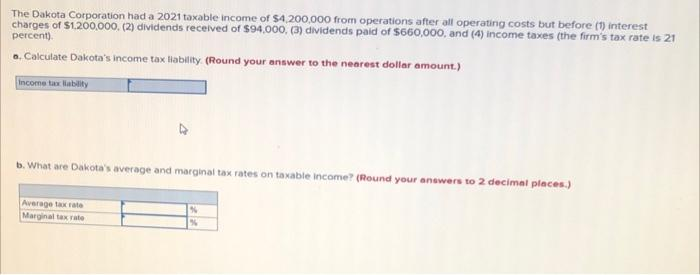# Question Solved1 AnswerThe Dakota Corporation had a 2021 taxable income of \$4,200,000 from operations after all operating costs but before (1) interest charges of \$1.200.000 (2) dividends received of \$94.000, (3) dividends paid of \$660,000, and (4) income taxes (the firm's tax rate is 21 percent) .. Calculate Dakota's income tax Hability (Round your answer to the nearest dollar amount.) income tax liability b. What are Dakota's average and marginal tax rates on taxable income? (Round your newers to 2 decimal places.) Average tax rate Marginal tax rateTranscribed Image Text: The Dakota Corporation had a 2021 taxable income of \$4,200,000 from operations after all operating costs but before (1) interest charges of \$1.200.000 (2) dividends received of \$94.000, (3) dividends paid of \$660,000, and (4) income taxes (the firm's tax rate is 21 percent) .. Calculate Dakota's income tax Hability (Round your answer to the nearest dollar amount.) income tax liability b. What are Dakota's average and marginal tax rates on taxable income? (Round your newers to 2 decimal places.) Average tax rate Marginal tax rate
More
Transcribed Image Text: The Dakota Corporation had a 2021 taxable income of \$4,200,000 from operations after all operating costs but before (1) interest charges of \$1.200.000 (2) dividends received of \$94.000, (3) dividends paid of \$660,000, and (4) income taxes (the firm's tax rate is 21 percent) .. Calculate Dakota's income tax Hability (Round your answer to the nearest dollar amount.) income tax liability b. What are Dakota's average and marginal tax rates on taxable income? (Round your newers to 2 decimal places.) Average tax rate Marginal tax rate